# Non-cooperative game

(diff) ← Older revision | Latest revision (diff) | Newer revision → (diff)

A systemwhere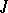is the set of players,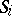is the set of strategies (cf. Strategy (in game theory)) of the-th player and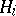is the gain function of the-th player, defined on the Cartesian product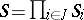. A non-cooperative game is played as follows: players, who are acting individually (do not form a coalition, do not cooperate), select their strategies, as a result of which the situation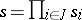appears, in which the-th player obtains the gain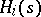. The main optimality principle in a non-cooperative game is the principle of realizability of the objective , which generates the Nash equilibrium solutions. A solutionis called an equilibrium solution if for all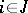,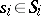, the inequalitywhere, is valid. Thus, none of the players is interested in unilaterally disturbing the equilibrium solution previously agreed upon between them. It has been proved (Nash's theorem) that a finite non-cooperative game (the setsandare finite) possesses an equilibrium solution for mixed strategies. This theorem has been generalized to include infinite non-cooperative games with a finite number of players  and non-cooperative games with an infinite number of players (cf. Non-atomic game).

Two equilibrium solutionsandare called interchangeable if any solution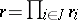, where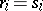or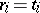,, is also an equilibrium solution. They are called equivalent if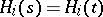for all. Letbe the set of all equilibrium solutions, and letbe the set of equilibrium solutions which are Pareto optimal (cf. Arbitration scheme). A game is called Nash solvable andis said to be a Nash solution if all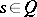are equivalent and interchangeable. A game is called strictly solvable if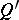is non-empty and all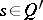are equivalent and interchangeable. Two-person zero-sum games (cf. Two-person zero-sum game) with optimal strategies are Nash solvable and strictly solvable; however, in the general case such a solvability is often impossible.

Other attempts at completing the principle of realizability of the objective were made. Thus, it was suggested  that the unique equilibrium solution or the maximum solution (in this last situation each player may ensure his/her own gain irrespective of the strategies chosen by the other players), the choice of which is based on the introduction of a new preference relation on the set of solutions, be considered as the solution of the non-cooperative game. In another approach the solution of a non-cooperative game is defined by a subjective prognosis of the behaviour of the players .

How to Cite This Entry:
Non-cooperative game. Encyclopedia of Mathematics. URL: http://encyclopediaofmath.org/index.php?title=Non-cooperative_game&oldid=17485
This article was adapted from an original article by E.I. VilkasE.B. Yanovskaya (originator), which appeared in Encyclopedia of Mathematics - ISBN 1402006098. See original article